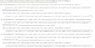# Imaginary numbers what to do with them

• larsbentley
I think I have found a workaround for my problem. I will post the solution in a separate topic.Thanks!In summary, Lars tried to solve for the peak velocity in a trapezoidal move where the following were already known: distance(d),total time(t),units of acceleration(Ma),units of deceleration(Ma),initial velocity(Vi), final velocity(always zero for my purposes). He found that one of the equations involved imaginary numbers and that he needed to use a different equation to get the correct answer.f

#### larsbentley

I have attached an image showing the three possible solutions (as determined by Mathematica) when solving for the peak velocity(Vs) in a trapezoidal move where the following are already known: distance(d),total time(t),units of acceleration(Ma),units of deceleration(Ma),initial velocity(Vi), final velocity(always zero for my purposes).

One of these equations is presumably correct, and you will notice that the second two equations contain an imaginary number 'i" in two places in the equation. I am trying to write the correct equation up in a programing language that has no provision for incorporating imaginary numbers.

Is there a way I can rewrite these equations without the 'i' imaginary number... substituting the 'i' with a placeholder of some kind and changing the negative values that need to be "square rooted" with positive ones?

I already tried writing up the first equation which uses no 'i' imaginary numbers in the programming language but the value under the square root turns out to be a negative number, causing an illegal square root error. If I simply change the value to positive the equation will process but it does not give a true answer based on the known values I input. I'm guessing that this is not the equation that will give me the actual solution, so I will need to use one of the two equations that involve imaginary numbers.

Can anyone help?

Thanks

#### Attachments

•speed formulas with ac dc counts2.jpg
41.3 KB · Views: 512
Before you spend too much effort, you should try plugging in numerical values using Mathematica, to make sure you actually have the answer you wanted!

What language are you using, by the way?

Hurkyl,

Good suggestion! None of the three formulas returned valid results when I plugged values back into them, but the source formula does produce valid results so I am not sure when I am doing wrong. As you can see in the attached jpg I get an error:

"Solve::ratnz: Solve was unable to solve the system with inexact coefficients. The answer was obtained by solving a corresponding exact system and numericizing the result"

Perhaps there is another method to solve for this equation in Mathematica, or I am writing it up incorrectly?

I have attached a new jpg showing that when I make assumptions about the values Mathematica will return the correct result as the third answer in its set of possible solutions.

#### Attachments

I have attached an image showing the three possible solutions (as determined by Mathematica) when solving for the peak velocity(Vs) in a trapezoidal move where the following are already known: distance(d),total time(t),units of acceleration(Ma),units of deceleration(Ma),initial velocity(Vi), final velocity(always zero for my purposes).

One of these equations is presumably correct, and you will notice that the second two equations contain an imaginary number 'i" in two places in the equation. I am trying to write the correct equation up in a programing language that has no provision for incorporating imaginary numbers.

Is there a way I can rewrite these equations without the 'i' imaginary number... substituting the 'i' with a placeholder of some kind and changing the negative values that need to be "square rooted" with positive ones?

I already tried writing up the first equation which uses no 'i' imaginary numbers in the programming language but the value under the square root turns out to be a negative number, causing an illegal square root error. If I simply change the value to positive the equation will process but it does not give a true answer based on the known values I input. I'm guessing that this is not the equation that will give me the actual solution, so I will need to use one of the two equations that involve imaginary numbers.

Can anyone help?

Thanks

I know that is possible to denote complex numbers with matrices instead of using i. That should be easy to find.

Thanks Patrick...

I'm going to create a new topic to try to solve the problem with my Mathematica output before continuing because I don't have a formula that works anyway.

Lars